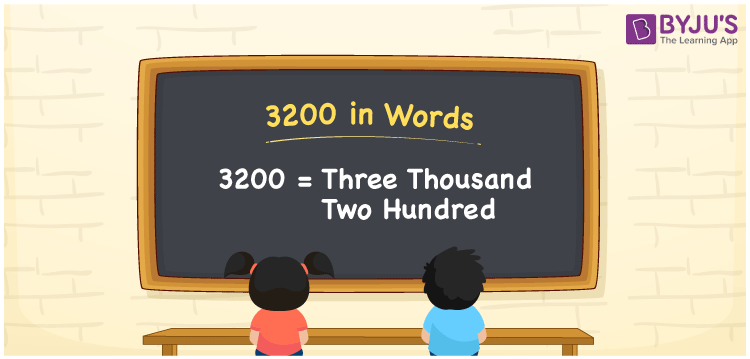# 3200 in Words

We can write 3200 in words as Three thousand two hundred. If you bought a new table at Rs. 3200, you can say, “I bought a new table that costs Three thousand two hundred rupees”. As we know, 3200 is a cardinal number as it denotes a specific amount.

 3200 in words Three thousand two hundred Three thousand two hundred in Numbers 3200

## 3200 in English Words

We generally write numbers in words using the English alphabet. Thus, we can read 3200 in English as “Three thousand two hundred”.## How to Write 3200 in Words?

Let us make a four-column place value chart to write the number 3200 in words since it is a four-digit number.

 Thousands Hundreds Tens Ones 3 2 0 0

Here, ones = 0, tens = 0, hundreds = 2, and thousands = 3.

Thus, we can write the expanded form as:

3 × Thousand + 2 × Hundred + 0 × Ten + 0 × One

= 3 × 1000 + 2 × 100 + 0 × 10 + 0 × 1

= 3000 + 200

= Three thousand + Two hundred

= Three thousand two hundred

Hence, 3200 in words is written as Three thousand two hundred.

3200 is a natural number that precedes 3201 and succeeds 3199.

3200 in words – Three thousand two hundred

Is 3200 an odd number? – No

Is 3200 an even number? – Yes

Is 3200 a prime number? – No

Is 3200 a composite number? – Yes

Is 3200 a perfect square number? – No

Is 3200 a perfect cube number? – No

## Frequently Asked Questions on 3200 in Words

Q1

### How do you say 3200 in words?

The spelling of 3200 in words is Three thousand two hundred, which means 3200 in words can be expressed as Three thousand two hundred.
Q2

### How to write 3200 rupees in words on a cheque?

On a cheque, we generally write 3200 rupees in words as Three thousand two hundred rupees only.
Q3

### What number is Three thousand two hundred in words?

The number 3200 is Three thousand two hundred in words, i.e. 3200 in words = Three thousand two hundred.